7608

Physics Vectors Level: Misc Level

A force vector has a magnitude 630 N and a direction of 45% north of east. Determine the magnitude and direction of the components of the force that point along the north -south line and along the east-west line.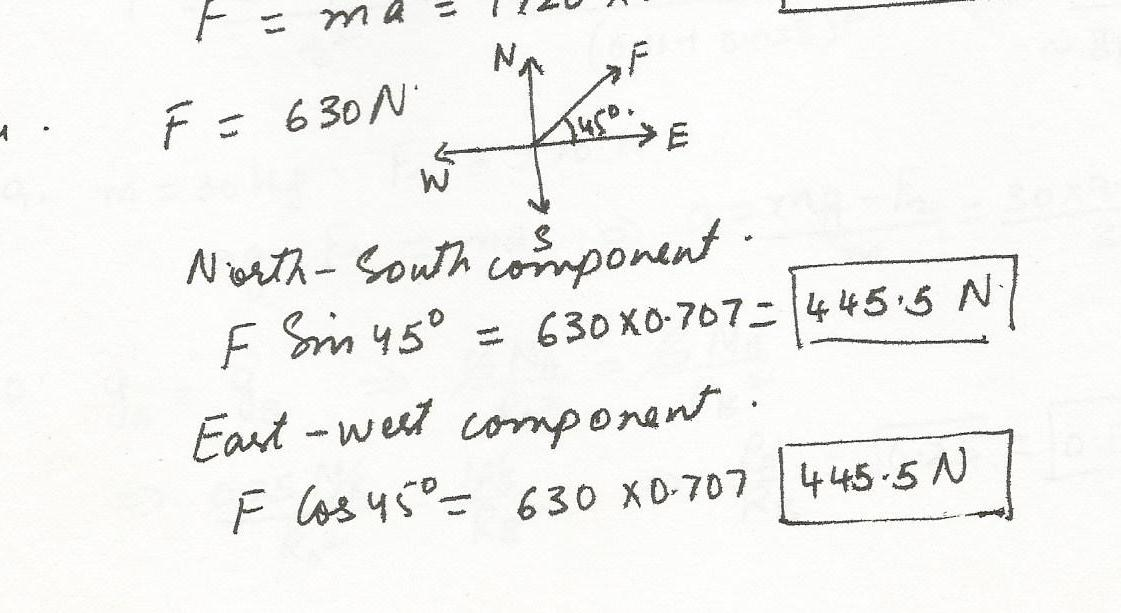7491

Physics Vectors Level: Misc Level

Three vectors A, B, and C add together to yield zero; A+B+C=0. The vectors A and C point in opposite directions and their magnitudes are related by the expression; A=2C. Which one of the following conclusions is correct?

a.A and B have equal magnitudes and point in opposite directions.

b.B and C have equal magnitudes and point in same direction.

c.B and C have equal magnitudes and point in opposite directions.

d.A and B point in the same direction, but A has twice the magnitude of B.

e. B and C point in the same direction, but C has twice the magnitude of B.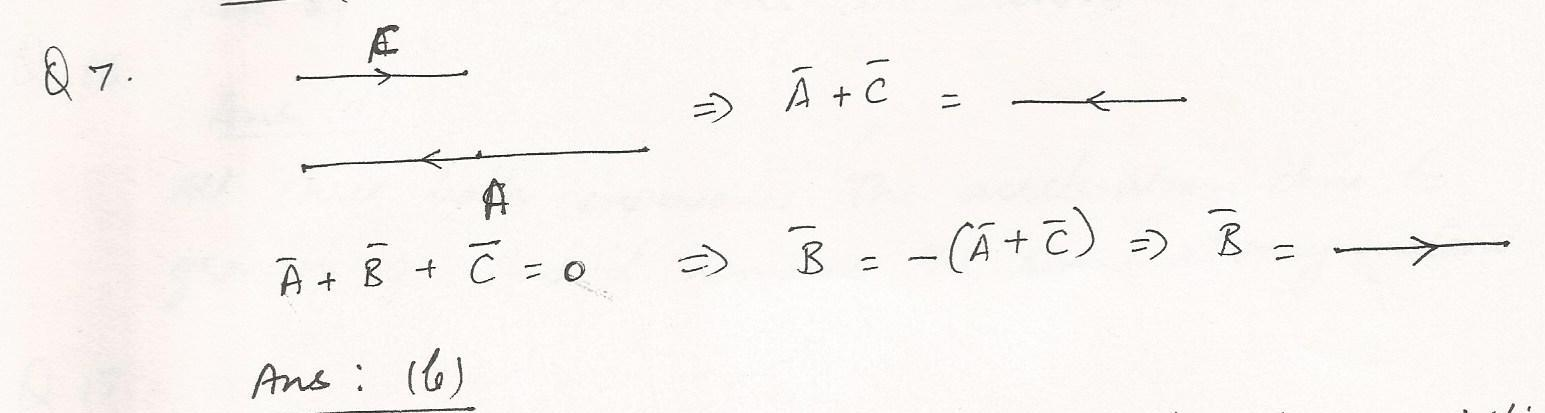7405

Physics Vectors Level: Misc Level

An Airplne is flying towards a destination 320 km due east of its starting point. There is a wind of 50 km/h from the northwest (towards bearing 135). The pilot wishes to make the trip in 40 minutes.What should his airspeed vector be?(The diagram is essential)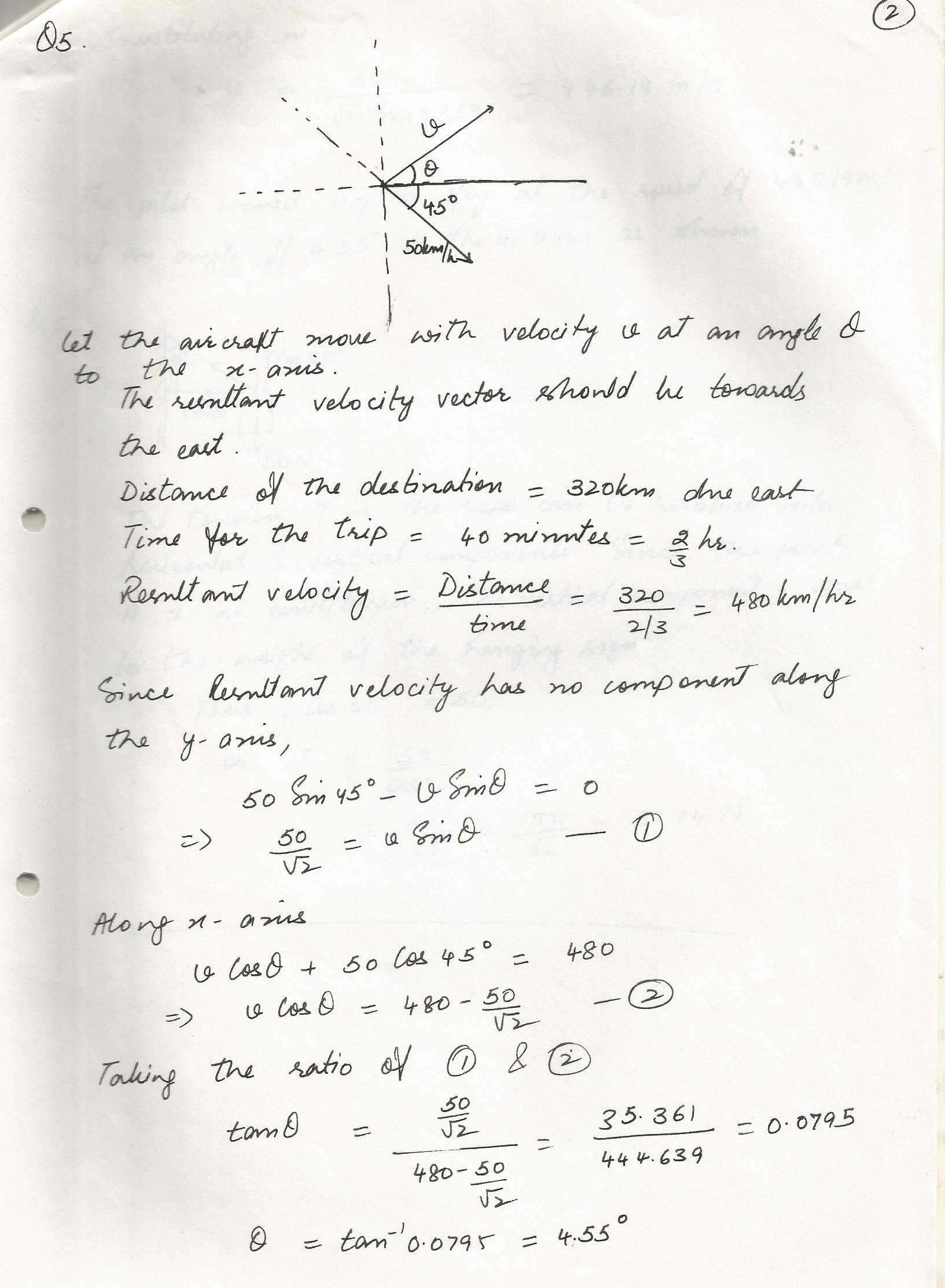7404

Physics Vectors Level: Misc Level

Sarah rows a boat across a river that is flowing at a steady 3 km/h. She keeps the boat pointing straight across, maintaining a rowing speed of 5km/h. The river is 1 km wide.

(a) From a vector diagram, find the actual crossing speed and direction of Sarah,s boat.

(b) Find the time it takes to cross the river,

(c) Find the distance down river that Sarah reaches the shore.7403

Physics Vectors Level: Misc Level

A rock of a weight 200N is resting on an incline which makes an angle of 30 degrees to the horizontal. Find the component of the weight force that makes the rock inclined to slide down the incline.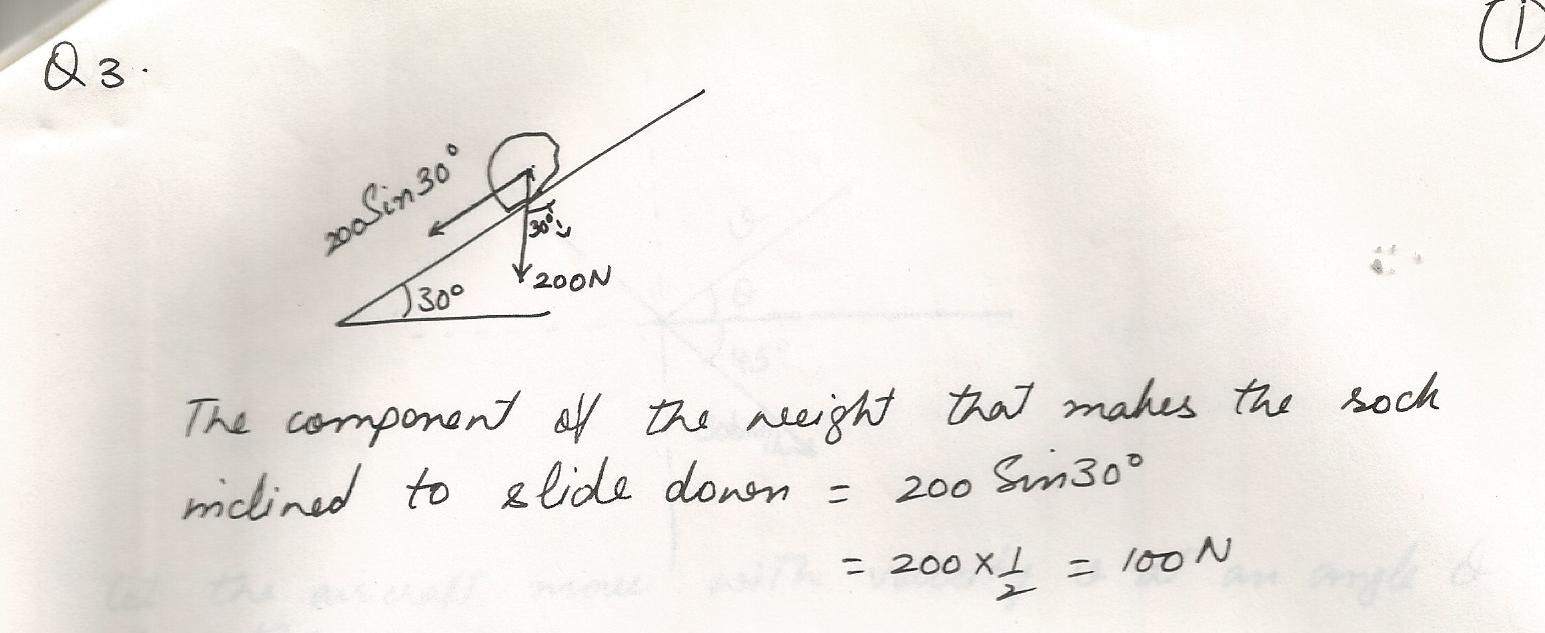7402

Physics Vectors Level: Misc Level

Given the vectors A (10 N.) and B (8N.) at an angle of 143 degrees to each other. Find the vector A-B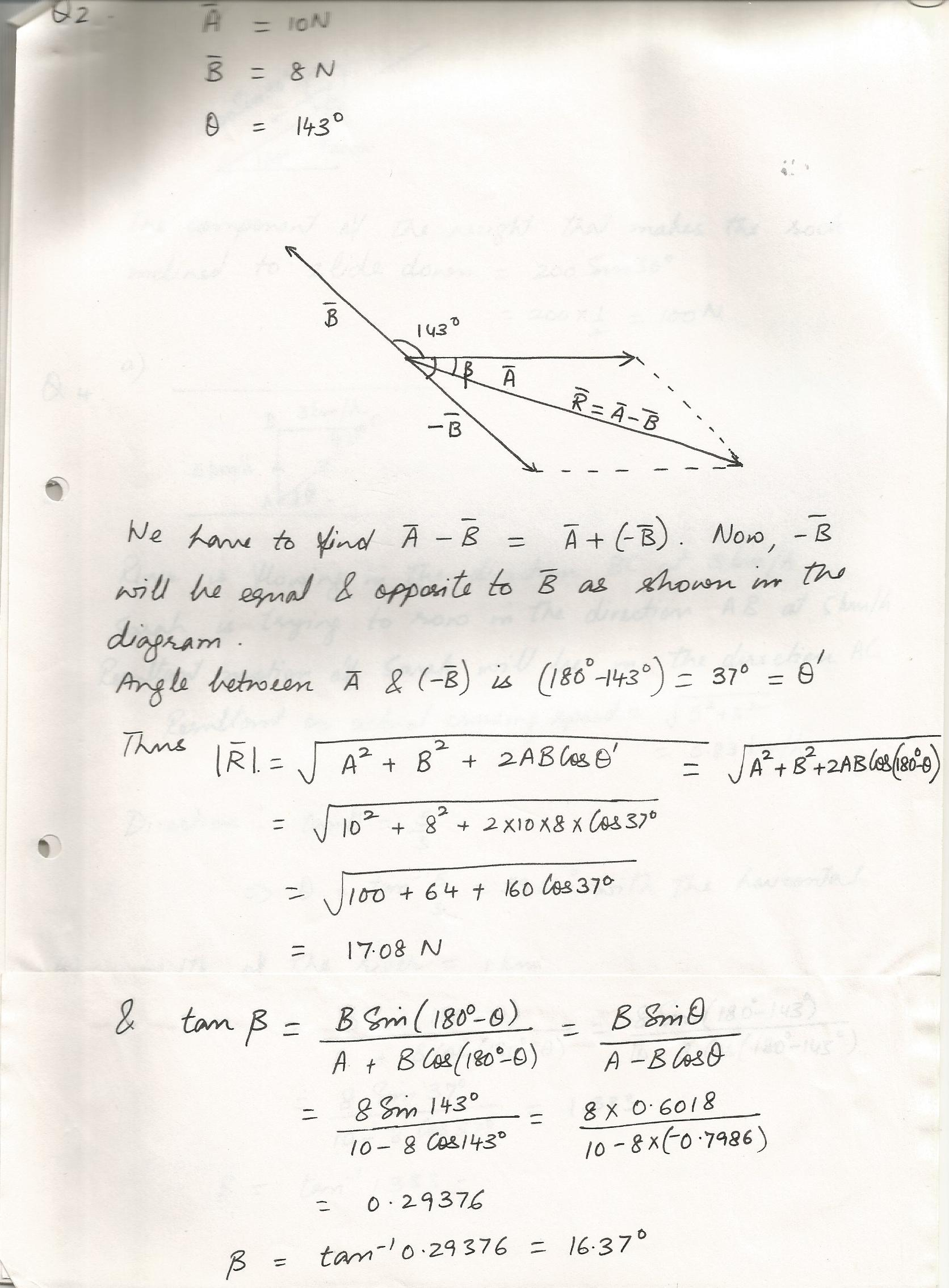7401

Physics Vectors Level: Misc Level

Find the resultant of two forces, 5N.and 6N. at an angle of 20 degrees to each other.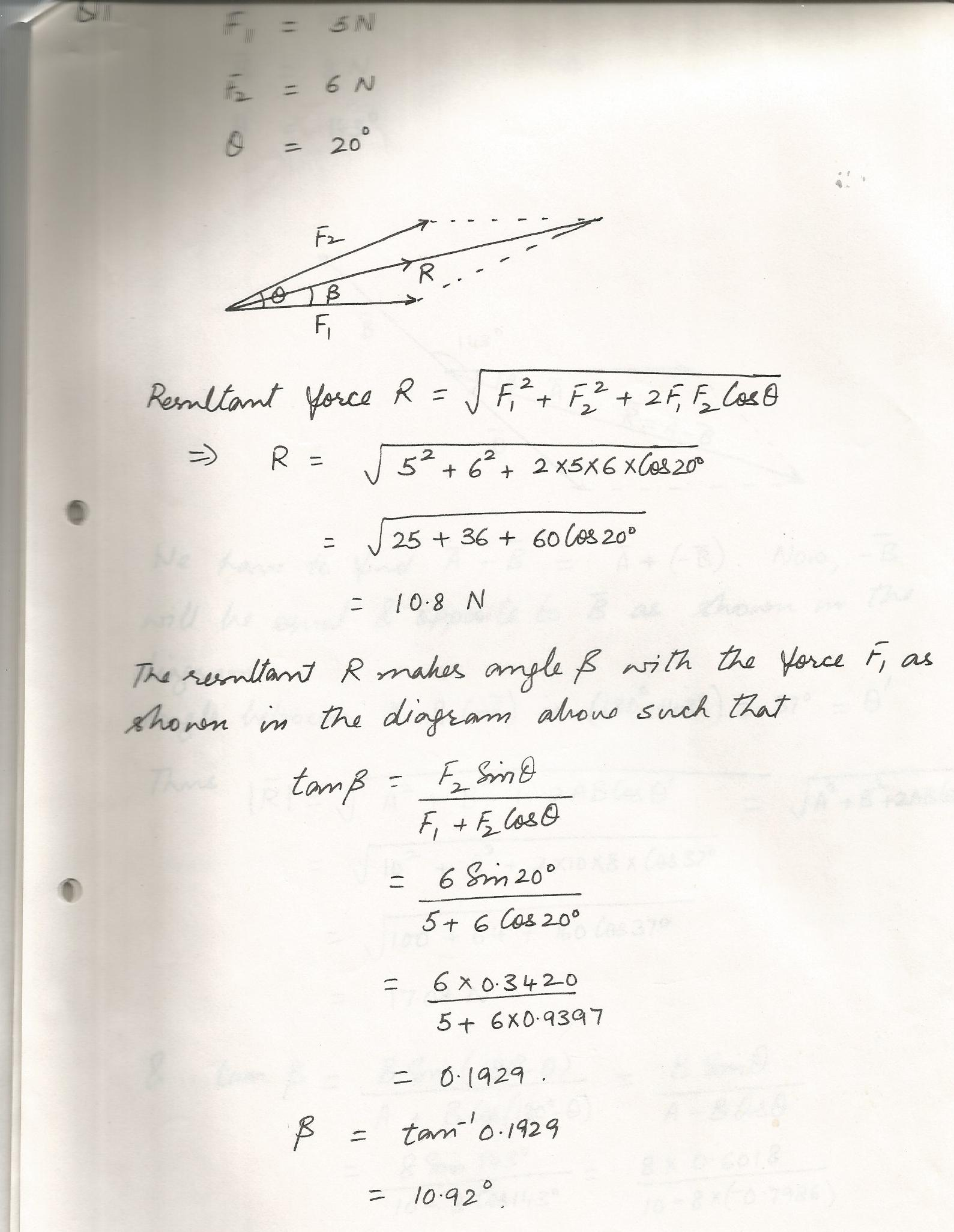6981

Physics Vectors Level: Misc Level

Joe must paddle a canoe such that he reaches camp at 30 degrees north of east, traveling at 10km/hr. However there is a current coming downstream at 5km/hr heading 45 degrees south of east.What will Joe,s water velocity and direction be in order to attain his intended velocity and direction?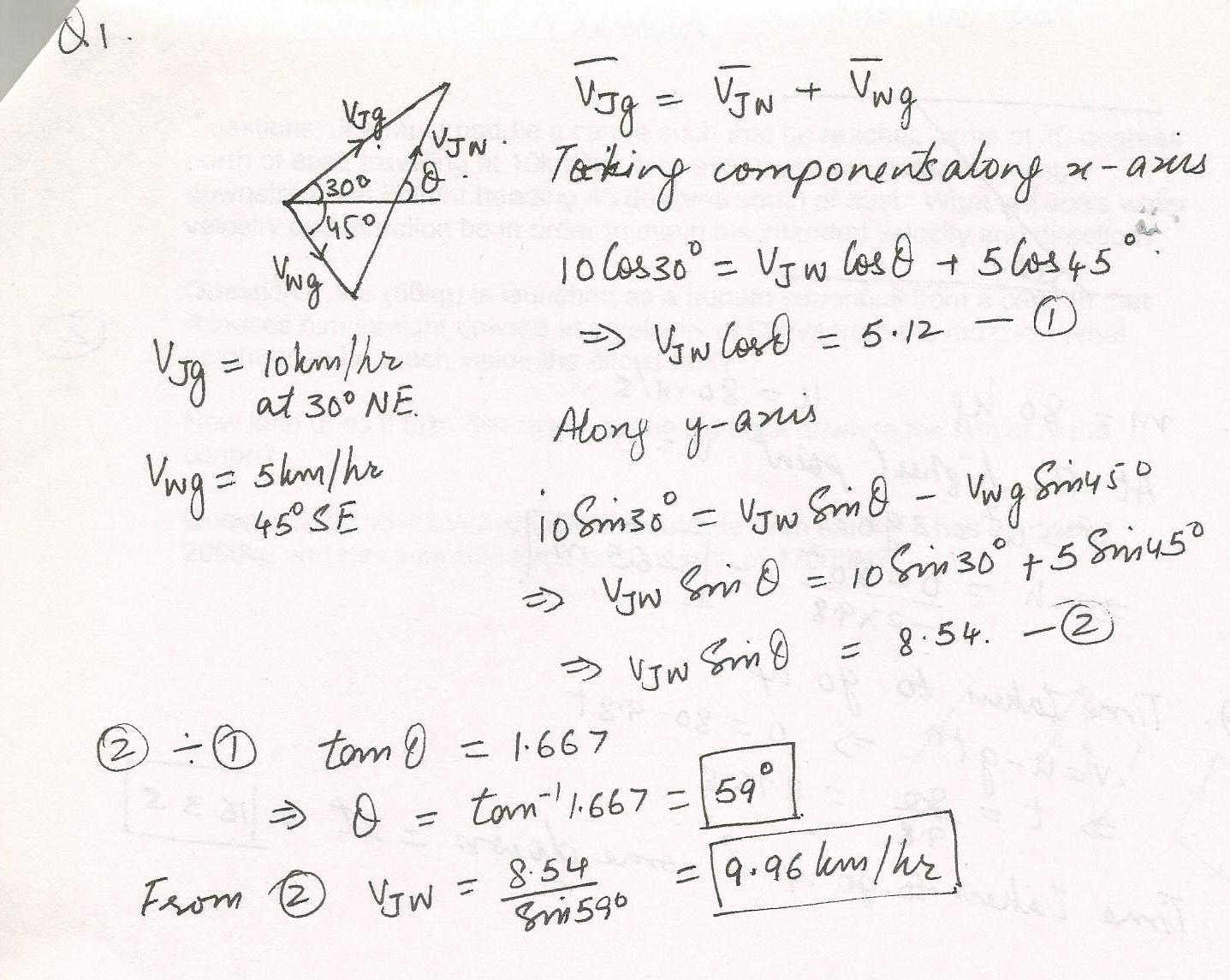6270

Physics Vectors Level: Misc Level

A person moves 30  m North, then 20 m East, and 30/2 m45% South of west. His displacement from the original position is:

a. 10 m West

b. 15 m East

c. 28 m South

d. 14 m South-West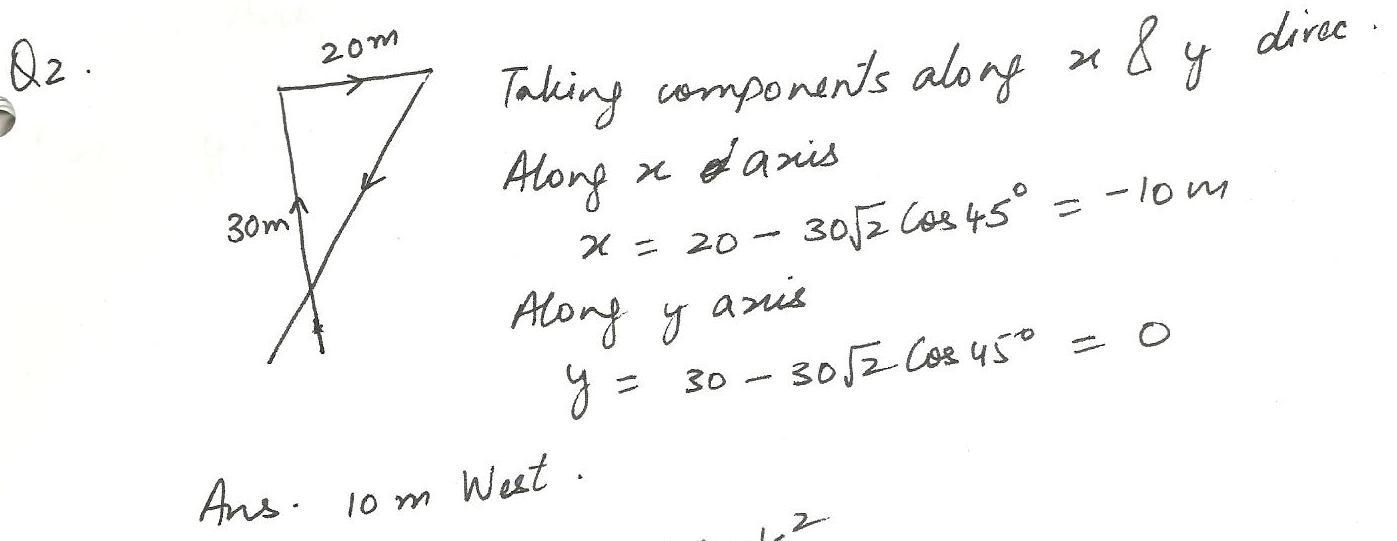6233

Physics Vectors Level: Misc Level

An object on by three forces moves with constant velocity. One force acting on the object is in the positive x direction and has a magnitude of 6.1 N: a second force has a magnitude of 4.1 N and points in the negative y direction. Find the direction and magnitude of the third force acting on the object. N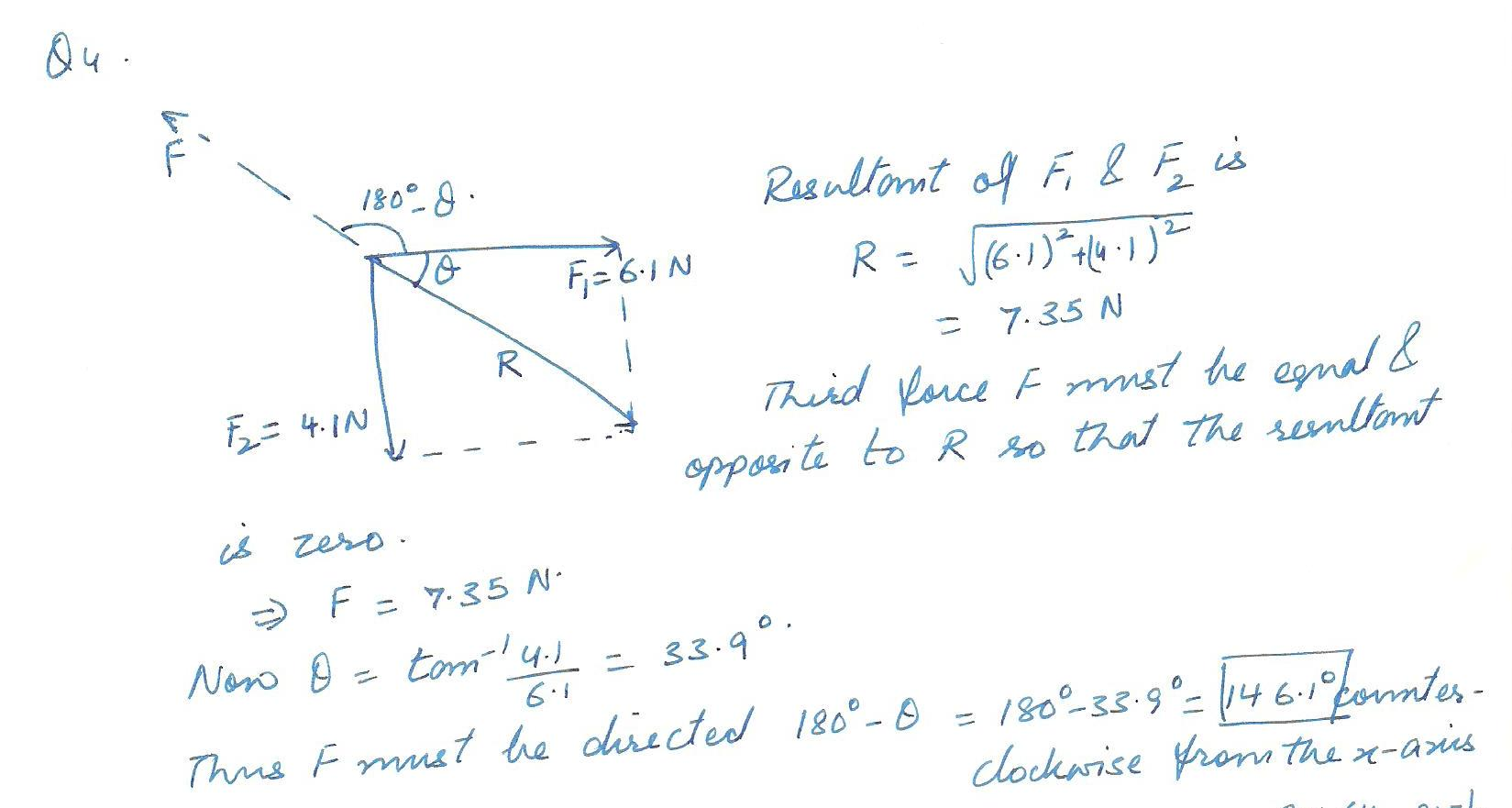6076

Physics Vectors Level: Misc Level

Sum of Three Vectors

The figure below shows three vectors of lengths A=68.5, B=40.4, and C=44.4. The angles are 0a=27.9% and 0b=55%, and C points along the negative y-axis.(a) Determine the length of the vector A-B+C.

(b) Calculate the angle of this vector.6074

Physics Vectors Level: Misc Level

The figure below shows three vectors of lengths A=63.9, B=38.4, and C=44.6.The angles are 0a=28.0% and 0b=54.9%, and Cpoints along the negative y-axis. (a) Determine the length of the vector A-C..

(b) Calculate the angle of this vector.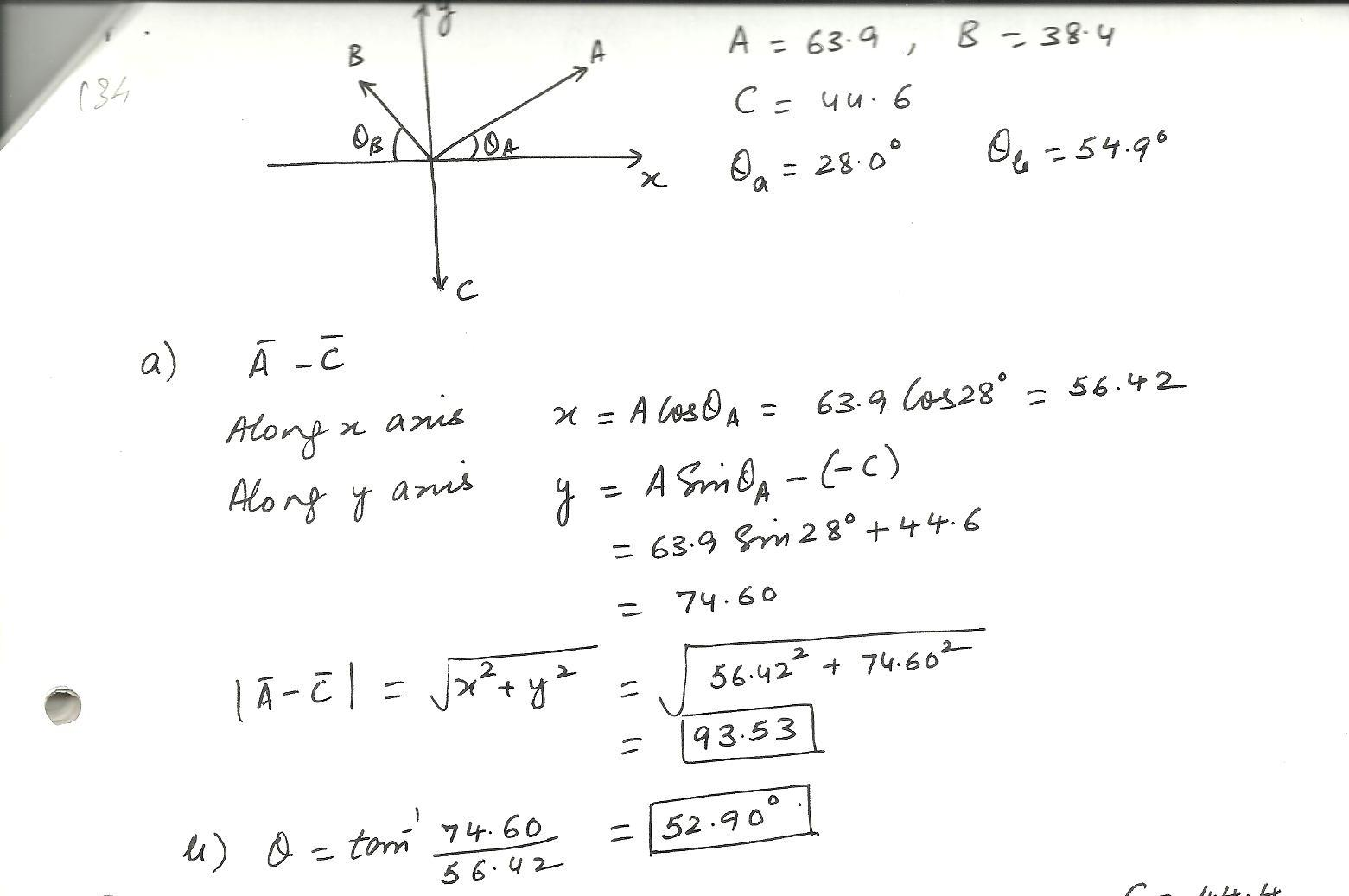6072

Physics Vectors Level: Misc Level

A basketball player runs down the court, following the path indicated by the vectors A,B,and C in the figure below. The magnitudes of these three vectors are A=11.1 m,B=22.8 m,and C=6.95 m. And as shown, 01=45.8% and 02=27.7% (a) Calculate the magnitude of the net displacement of this player.

b) What is the direction of the displacement of this player? Give the angle counterclockwise relative to the right.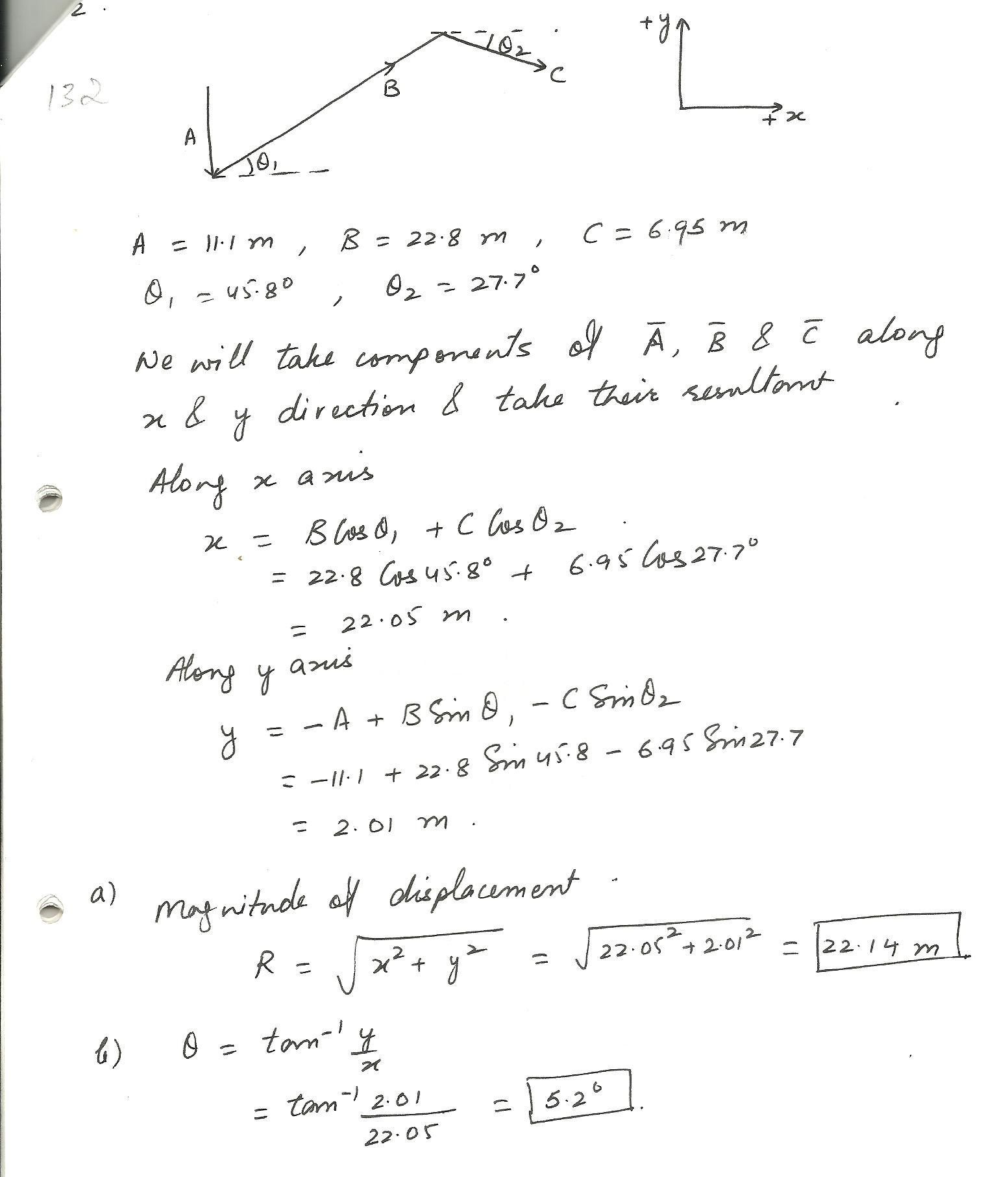6071

Physics Vectors Level: Misc Level

a) If Vx=19.40 units and Vy=-15.80 units, determine the magnitude of V.

b) Determine the angle of V with respect to the x-axis.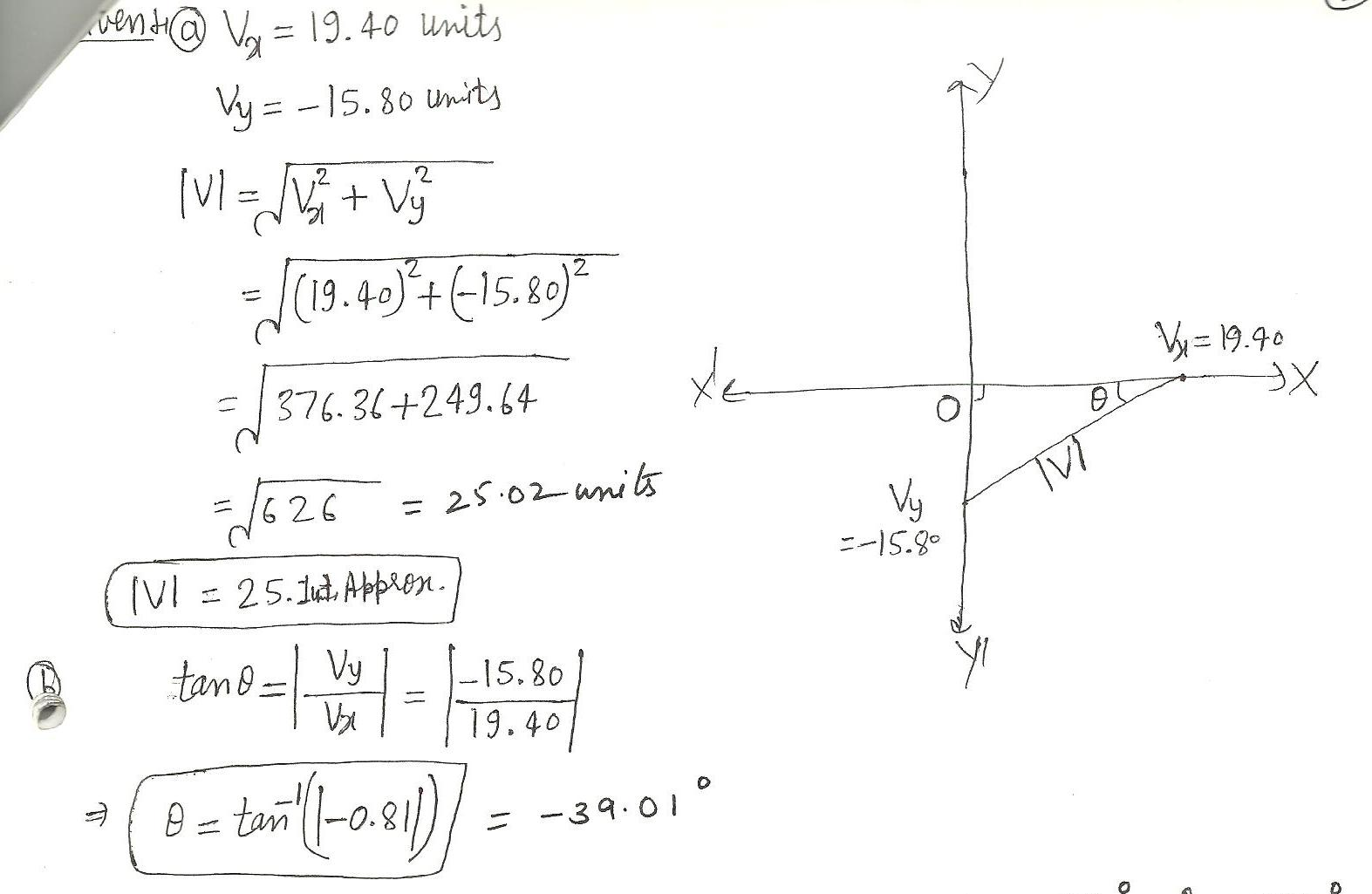5894

Physics Vectors Level: Misc Level

3.55 The velocity of the wind relative to the water is crucial to sailboats. Suppose a sailboat is in an ocean current that has a velocity of 2.20 m/s in a direction 30 degrees east of north realtive to the earth.It encounters a wind that has a velocity of 4.50 m/s in a direction of 50 degrees south of west relative to the earth. What is the velocity of the wind relative to the water?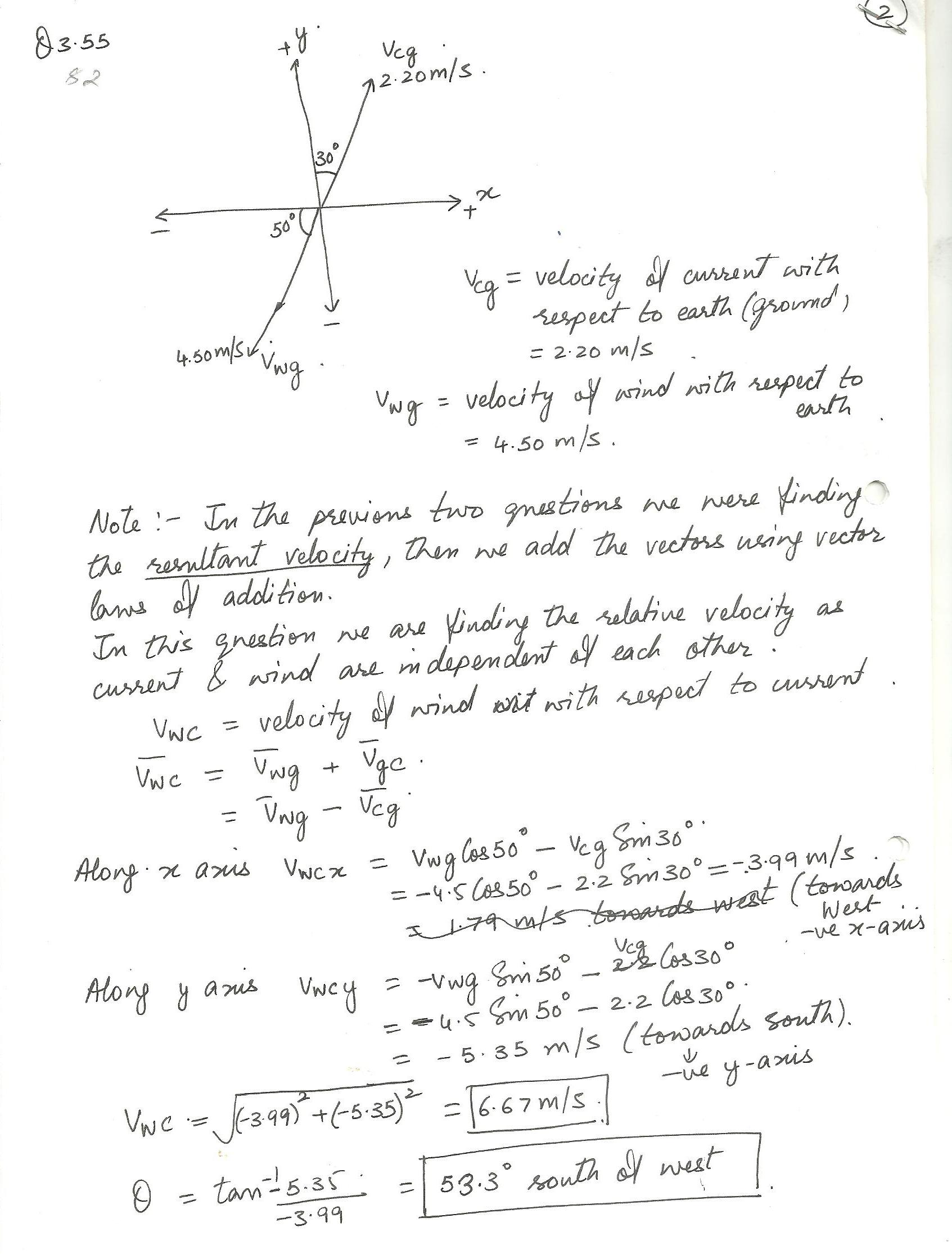Displaying 1-15 of 84 results.